SSC  >  Fluid Mechanics - 1

# Fluid Mechanics - 1

Test Description

## 20 Questions MCQ Test Mock Test Series for SSC JE Mechanical Engineering | Fluid Mechanics - 1

Fluid Mechanics - 1 for SSC 2022 is part of Mock Test Series for SSC JE Mechanical Engineering preparation. The Fluid Mechanics - 1 questions and answers have been prepared according to the SSC exam syllabus.The Fluid Mechanics - 1 MCQs are made for SSC 2022 Exam. Find important definitions, questions, notes, meanings, examples, exercises, MCQs and online tests for Fluid Mechanics - 1 below.
Solutions of Fluid Mechanics - 1 questions in English are available as part of our Mock Test Series for SSC JE Mechanical Engineering for SSC & Fluid Mechanics - 1 solutions in Hindi for Mock Test Series for SSC JE Mechanical Engineering course. Download more important topics, notes, lectures and mock test series for SSC Exam by signing up for free. Attempt Fluid Mechanics - 1 | 20 questions in 12 minutes | Mock test for SSC preparation | Free important questions MCQ to study Mock Test Series for SSC JE Mechanical Engineering for SSC Exam | Download free PDF with solutions
 1 Crore+ students have signed up on EduRev. Have you?
Fluid Mechanics - 1 - Question 1

### What is the specific gravity of a fluid whose specific weight is 7.85 kN/m3?

Detailed Solution for Fluid Mechanics - 1 - Question 1

Specific Weight:

$$\gamma = \rho g = 7.85 \Rightarrow \rho = \frac{{7.85 \times {{10}^3}}}{{10}} = 785$$

Specific gravity:

$$s = \frac{\rho }{{{\rho _{water}}}} = \frac{{785}}{{1000}} = 0.8$$

Fluid Mechanics - 1 - Question 2

### A pump delivers water at a rate of 0.025 m3/s against a head of 30 m. If the overall efficiency of the pump is 75%, the power required by the pump is:

Detailed Solution for Fluid Mechanics - 1 - Question 2

P=ρgQH=1000×9.81×0.025×30=7357.5WP=ρgQH=1000×9.81×0.025×30=7357.5W

Power required: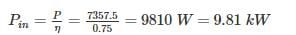Fluid Mechanics - 1 - Question 3

### The velocity potential which follow the equation of continuity is ________

Detailed Solution for Fluid Mechanics - 1 - Question 3

Velocity Potential function (ϕ) is given as = x2 - y2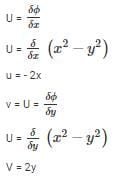For continuity to be satisfied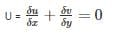-2 + 2 = 0

(Hence satisfied)

Fluid Mechanics - 1 - Question 4

When a liquid rotates at a constant angular velocity about a vertical axis as a rigid body, the pressure

Detailed Solution for Fluid Mechanics - 1 - Question 4

A fluid is rotating at constant angular velocity ω about the central vertical axis of a cylindrical container. The variation of pressure in the radial direction is given by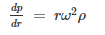It is given that the pressure at the axis of rotation is Pc.

Therefore, the required pressure at any point r is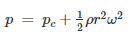Fluid Mechanics - 1 - Question 5

Pressure of 200 kPa is equivalent to the head of z metre of liquid having relative density 1.59. The value of z (m) is _____.

Detailed Solution for Fluid Mechanics - 1 - Question 5

P = ρgh

h = z

P = ρgz

200 × 103 = 1.59 × 1000 × 9.81 × z

z = 12.82 metres

Where P = Pressure (pascal)

ρ = density of fluid (kg/m3)

g = acceleration due to gravity (m/s2)

h = height of liquid column (metres)

Fluid Mechanics - 1 - Question 6

Which of the following is CORRECT about the viscosity of gas?

Detailed Solution for Fluid Mechanics - 1 - Question 6

As temperature increases, randomness in molecules also increases. This increase in randomness will result in increase in viscosity as viscosity of gas depends on randomness & collision of gas molecules.

Phenomenon: As a gas is heated, the movement of gas molecules increases and the probability that one gas molecule will collide with another gas molecule increases. In other words, increasing gas temperature causes the gas molecules to collide more often. This increases the gas viscosity because the transfer of momentum between stationary and moving molecules is what causes gas viscosity.

Fluid Mechanics - 1 - Question 7

The barometric pressure at the base of a mountain is 750 mm Hg and at the top 600 mm Hg. if the average air density is 1 kg/m3, the height of the mountain (in m) is approximately

Detailed Solution for Fluid Mechanics - 1 - Question 7

At base PB = 750 mm of Hg

At top, PA = 600 mm of Hg

∴ Pressure difference = 150 mm of Hg

(ΔP) = hρg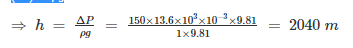⇒ Height of mountain = 2040 m

Fluid Mechanics - 1 - Question 8

Runaway speed of a hydraulic turbine is

Detailed Solution for Fluid Mechanics - 1 - Question 8

The runaway speed of a water turbine is its speed at full flow, and no shaft load. The turbine will be designed to survive the mechanical forces of this speed.

Fluid Mechanics - 1 - Question 9

The centre of pressure on inclined immersed surface remains valid for the centre of pressure on vertical immersed surface, if the angle (θ) at immersed surface with liquid surface is:

Detailed Solution for Fluid Mechanics - 1 - Question 9

Centre of Pressure on a Immersed surface from water surface is given by: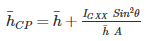For vertical surface θ = 90°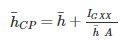So, the formula is valid for θ = 90°

Fluid Mechanics - 1 - Question 10

The flow in which the velocity vector is identical in magnitude and direction at every point, for any given instant, is known as

Detailed Solution for Fluid Mechanics - 1 - Question 10

The flow is defined as uniform flow when in the flow field the velocity and other hydrodynamic parameters do not change from point to point at any instant of time. For a uniform flow, there will be no spatial distribution of hydrodynamic and other parameters.

When the velocity and other hydrodynamic parameters changes from one point to another the flow is defined as non-uniform.

steady flow is defined as a flow in which the various hydrodynamic parameters and fluid properties at any point do not change with time.

One-dimensional flow is the flow where all the flow parameters may be expressed as functions of time and one space coordinate only. The single space coordinate is usually the distance measured along the centre-line (not necessarily straight) in which the fluid is flowing. Example: the flow in a pipe is considered one - dimensional when variations of pressure and velocity occur along the length of the pipe, but any variation over the cross-section is assumed negligible.

Turbulent fluid motion can be considered as an irregular condition of flow in which various quantities (such as velocity components and pressure) show a random variation with time and space.

Fluid Mechanics - 1 - Question 11

Select the correct option for the velocity profile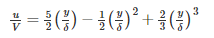Detailed Solution for Fluid Mechanics - 1 - Question 11

The separation point S is determined from the condition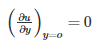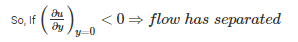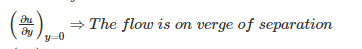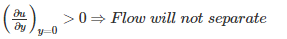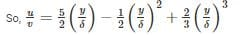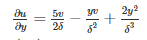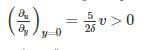⇒ Flow will not separate.

Fluid Mechanics - 1 - Question 12

In Kaplan turbine runner, the number of blades is generally of the order _____.

Detailed Solution for Fluid Mechanics - 1 - Question 12

Kaplan turbine has adjustable runner blades. Kaplan Turbine has very small number of blades 3 to 8. France Turbine has very large number of blades 16 to 24

Fluid Mechanics - 1 - Question 13

ln a two-dimensional flow, where u is the x-component and v is the y-component of velocity the equation of streamline is given by

Detailed Solution for Fluid Mechanics - 1 - Question 13

Equation of stream line is given as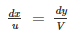⇒ Vdx – udy = 0

Fluid Mechanics - 1 - Question 14

Kinematic viscosity of air at 20°C is given to be 1.6 × 10-5 m2/s. Its kinematic viscosity at 70°C will be approximately

Detailed Solution for Fluid Mechanics - 1 - Question 14

Dynamic viscosity of gases increase with temp μ∝T−−√μ∝T

Density of gases decreases with increase in temp ρ∝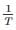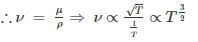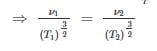T1 = 20 + 273 = 293 K   T2 = 70 + 273 = 343 K
ν1 = 1.6 × 10-5 m2/s       ν2 = ?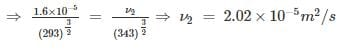Fluid Mechanics - 1 - Question 15

The equation gz+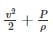constant along a stream line holds true for

Detailed Solution for Fluid Mechanics - 1 - Question 15

PdA - (P + dP) dA - ρgdAds sin θ = 0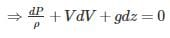⇒dPρ+VdV+gdz=0⇒dPρ+VdV+gdz=0

Assumptions in Bernoulli’s equation:

i) fluid is ideal

ii) flow is steady

iii) flow is continuous

iv) fluid is incompressible

v) flow is non-viscous

vi) flow is irrotational

vii) applicable along a stream line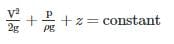where,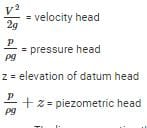• The line representing the sum of all 3 head is known as total energy line or total head line
• Line joining the points of piezometric heads is known as hydraulic grade line or piezometric line.
Fluid Mechanics - 1 - Question 16

If for a fluid in motion, the pressure at a point is same in all directions, then the fluid is

Detailed Solution for Fluid Mechanics - 1 - Question 16

An ideal fluid is a fluid that has several properties including the fact that it is:

Incompressible - the density is constant with respect to pressure.

Irrotational - the flow is smooth, no turbulence.

Nonviscous - (Inviscid) fluid has no internal friction (η = 0)

Fluid Mechanics - 1 - Question 17

A fully developed laminar viscous flow through a circular tube has the ratio of maximum velocity to average velocity as

Detailed Solution for Fluid Mechanics - 1 - Question 17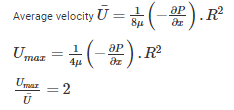Fluid Mechanics - 1 - Question 18

The diameter of a soap bubble which has an inside pressure of 2.5 N/mover the atmospheric pressure and a surface tension of 0.0125 N/m is

Detailed Solution for Fluid Mechanics - 1 - Question 18

For soap bubble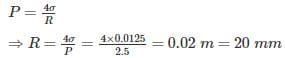∴ Diameter = 40 mm

Fluid Mechanics - 1 - Question 19

In Bernoulli’s equation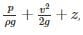each term represents:

Detailed Solution for Fluid Mechanics - 1 - Question 19

If the expression is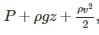then it has units of total energy per unit volume.

The expression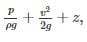, has units of energy per unit weight.

Fluid Mechanics - 1 - Question 20

A fluid when acted upon by a shear stress will deform

Detailed Solution for Fluid Mechanics - 1 - Question 20

Viscosity is the property of the fluid to resist the rate at which deformation takes place when the fluid is acted upon by shear force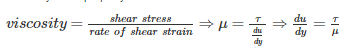A fluid when acted upon by a shear stress will deform when the applied shear stress is greater than the viscous strength of the fluid.

## Mock Test Series for SSC JE Mechanical Engineering

3 videos|1 docs|55 tests
 Use Code STAYHOME200 and get INR 200 additional OFF Use Coupon Code
Information about Fluid Mechanics - 1 Page
In this test you can find the Exam questions for Fluid Mechanics - 1 solved & explained in the simplest way possible. Besides giving Questions and answers for Fluid Mechanics - 1, EduRev gives you an ample number of Online tests for practice

## Mock Test Series for SSC JE Mechanical Engineering

3 videos|1 docs|55 tests